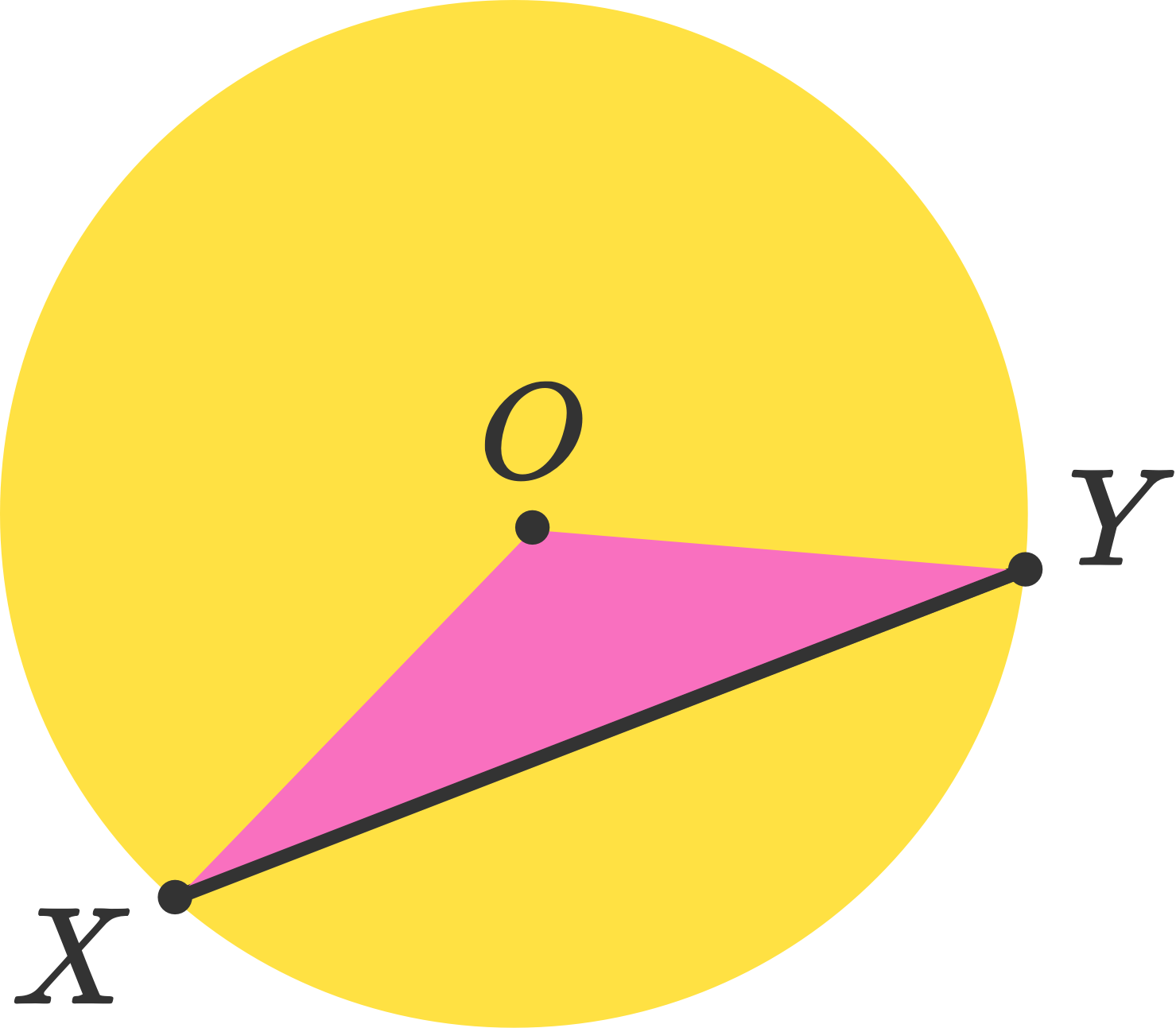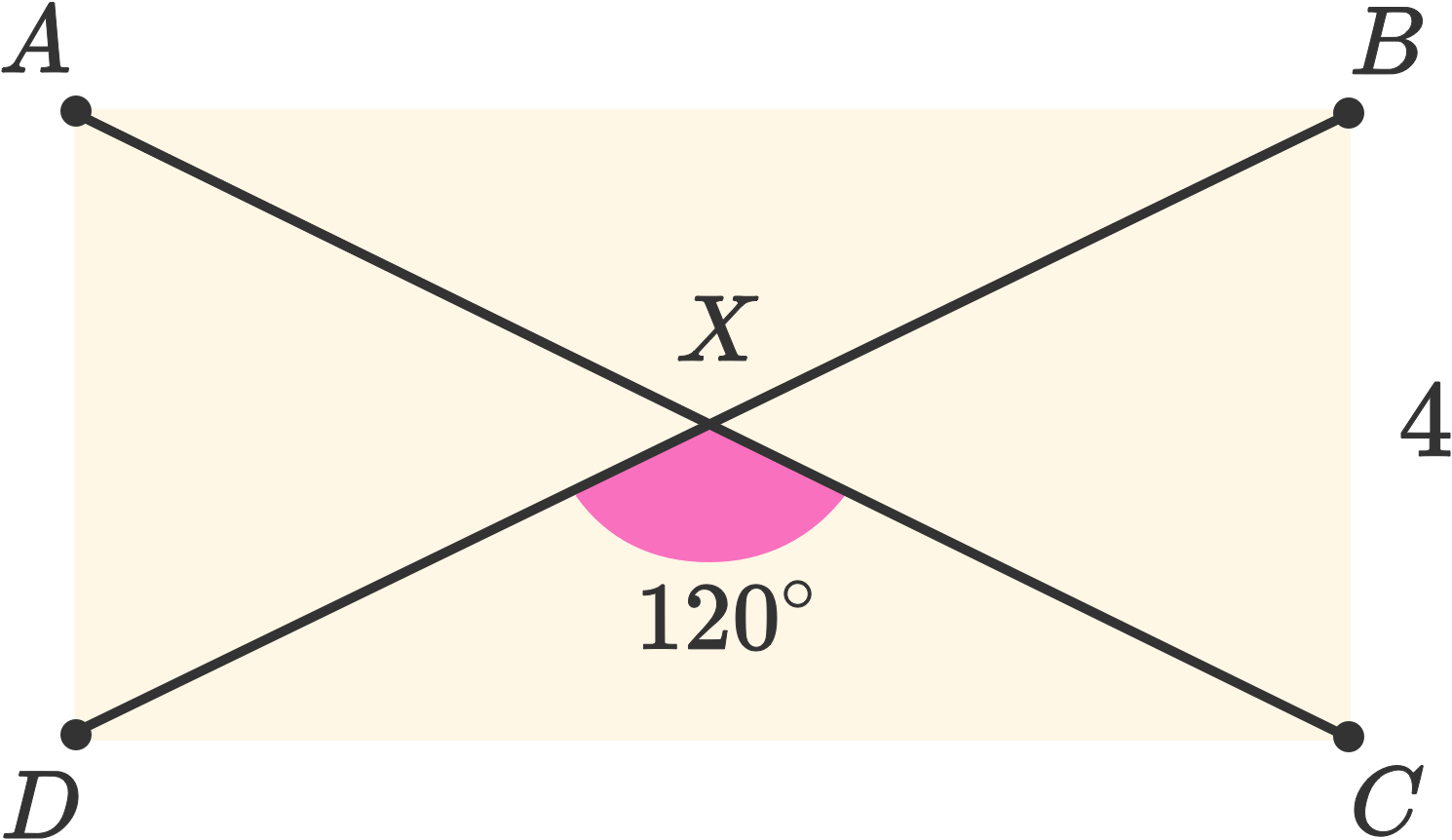Geometry

# Geometry Competition WarmupThe area of a right triangle is 30. One of the legs is 2 units more than 2 times the other. What is the hypotenuse of the triangle?Two equilateral triangles are aligned on top of one another to create a regular hexagon. If the area of the hexagon is 24, what is the area of one of the original equilateral triangles?Circle $O$ has point $X$ and point $Y$ on its circumference. If the radius of the circle is 6 and the measure of $XY$ is 10, what is the area of $\triangle{XOY}?$

The ratio of acute angle $A$ to acute angle $B$ is 7 to 4. The complement of one angle is $15^\circ$ larger than the complement of the other. What is $A + B?$The diagonals of rectangle $ABCD$ meet at point $X.$ $\angle {DXC} = 120^\circ.$ If the shorter side of the rectangle measures 4 units, what is the perimeter of the rectangle?

×